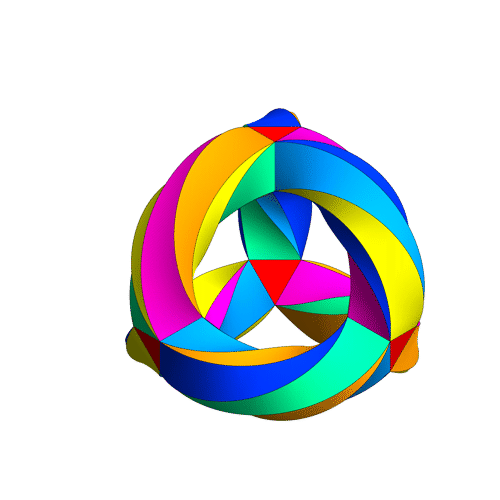## April 30, 2016

### Relative Endomorphisms

#### Posted by Qiaochu YuanLet $(M, \otimes)$ be a monoidal category and let $C$ be a left module category over $M$, with action map also denoted by $\otimes$. If $m \in M$ is a monoid and $c \in C$ is an object, then we can talk about an action of $m$ on $c$: it’s just a map

$\alpha : m \otimes c \to c$

satisfying the usual associativity and unit axioms. (The fact that all we need is an action of $M$ on $C$ to define an action of $m$ on $c$ is a cute instance of the microcosm principle.)

This is a very general definition of monoid acting on an object which includes, as special cases (at least if enough colimits exist),

• actions of monoids in $\text{Set}$ on objects in ordinary categories,
• actions of monoids in $\text{Vect}$ (that is, algebras) on objects in $\text{Vect}$-enriched categories,
• actions of monads (letting $M = \text{End}(C)$), and
• actions of operads (letting $C$ be a symmetric monoidal category and $M$ be the monoidal category of symmetric sequences under the composition product)

This definition can be used, among other things, to straightforwardly motivate the definition of a monad (as I did here): actions of a monoidal category $M$ on a category $C$ correspond to monoidal functors $M \to \text{End}(C)$, so every action in the above sense is equivalent to an action of a monad, namely the image of the monoid $m$ under such a monoidal functor. In other words, monads on $C$ are the universal monoids which act on objects $c \in C$ in the above sense.

Corresponding to this notion of action is a notion of endomorphism object. Say that the relative endomorphism object $\text{End}_M(c)$, if it exists, is the universal monoid in $M$ acting on $c$: that is, it’s a monoid acting on $c$, and the action of any other monoid on $c$ uniquely factors through it.

Posted at 1:40 AM UTC | Permalink | Followups (9)

## April 23, 2016

### Polygonal Decompositions of Surfaces

#### Posted by John BaezIf you tell me you’re going to take a compact smooth 2-dimensional manifold and subdivide it into polygons, I know what you mean. You mean something like this picture by Norton Starr:or this picture by Greg Egan:(Click on the images for details.) But what’s the usual term for this concept, and the precise definition? I’m writing a paper that uses this concept, and I don’t want to spend my time proving basic stuff. I want to just refer to something.

Posted at 4:54 PM UTC | Permalink | Followups (14)

## April 21, 2016

### Type Theory and Philosophy at Kent

#### Posted by David CorfieldI haven’t been around here much lately, but I would like to announce this workshop I’m running on 9-10 June, Type Theory and Philosophy. Following some of the links there will show, I hope, the scope of what may be possible.

One link is to the latest draft of an article I’m writing, Expressing ‘The Structure of’ in Homotopy Type Theory, which has evolved a little over the year since I posted The Structure of A.